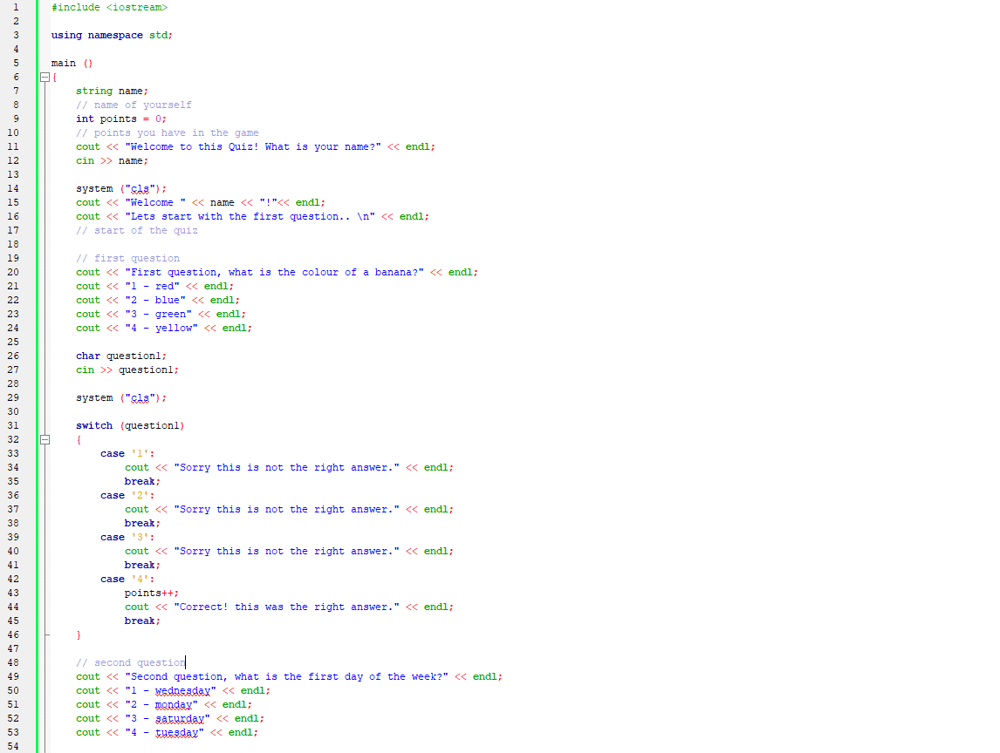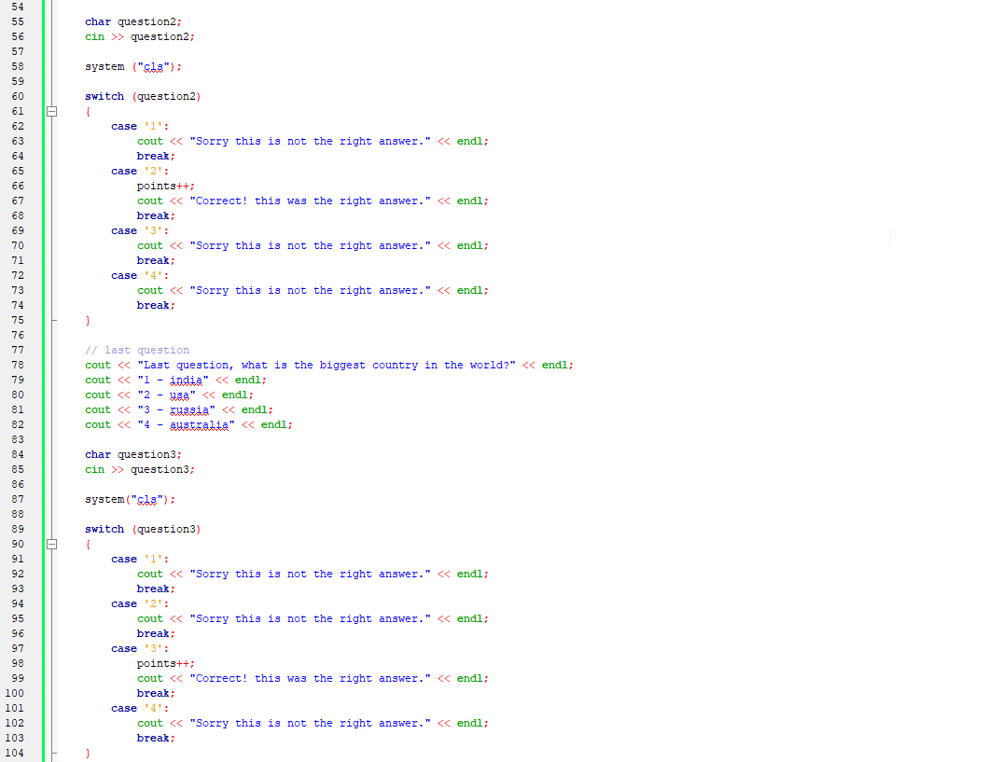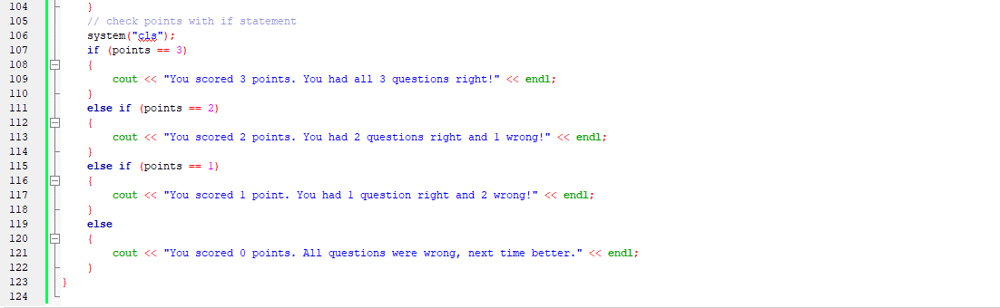## quiz

I wanted to try something different other than a calculator with the same things I've learned so far. Let me know what you think, it's quite basic I suppose.#include <iostream>

using namespace std;

main ()
{
string name;
// name of yourself
int points = 0;
// points you have in the game
cout << "Welcome to this Quiz! What is your name?" << endl;
cin >> name;

system ("cls");
cout << "Welcome " << name << "!"<< endl;
// start of the quiz

// first question
cout << "First question, what is the colour of a banana?" << endl;
cout << "1 - red" << endl;
cout << "2 - blue" << endl;
cout << "3 - green" << endl;
cout << "4 - yellow" << endl;

char question1;
cin >> question1;

system ("cls");

switch (question1)
{
case '1':
cout << "Sorry this is not the right answer." << endl;
break;
case '2':
cout << "Sorry this is not the right answer." << endl;
break;
case '3':
cout << "Sorry this is not the right answer." << endl;
break;
case '4':
points++;
cout << "Correct! this was the right answer." << endl;
break;
}

// second question
cout << "Second question, what is the first day of the week?" << endl;
cout << "1 - wednesday" << endl;
cout << "2 - monday" << endl;
cout << "3 - saturday" << endl;
cout << "4 - tuesday" << endl;

char question2;
cin >> question2;

system ("cls");

switch (question2)
{
case '1':
cout << "Sorry this is not the right answer." << endl;
break;
case '2':
points++;
cout << "Correct! this was the right answer." << endl;
break;
case '3':
cout << "Sorry this is not the right answer." << endl;
break;
case '4':
cout << "Sorry this is not the right answer." << endl;
break;
}

// last question
cout << "Last question, what is the biggest country in the world?" << endl;
cout << "1 - india" << endl;
cout << "2 - usa" << endl;
cout << "3 - russia" << endl;
cout << "4 - australia" << endl;

char question3;
cin >> question3;

system("cls");

switch (question3)
{
case '1':
cout << "Sorry this is not the right answer." << endl;
break;
case '2':
cout << "Sorry this is not the right answer." << endl;
break;
case '3':
points++;
cout << "Correct! this was the right answer." << endl;
break;
case '4':
cout << "Sorry this is not the right answer." << endl;
break;
}
// check points with if statement
system("cls");
if (points == 3)
{
cout << "You scored 3 points. You had all 3 questions right!" << endl;
}
else if (points == 2)
{
cout << "You scored 2 points. You had 2 questions right and 1 wrong!" << endl;
}
else if (points == 1)
{
cout << "You scored 1 point. You had 1 question right and 2 wrong!" << endl;
}
else
{
cout << "You scored 0 points. All questions were wrong, next time better." << endl;
}
}